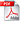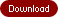# R˛ - Heaps with Suspended Relaxation for Manipulating Priority Queues and a New Algorithm for Reweighting Graphs

### by Ruth ShrairmanView First 25 Pages:(free download)

#### Synopsis

This research is dedicated to two main problems in finding shortest paths in the graphs. The first problem is to find shortest paths from an origin to all other vertices in non-negatively weighted graph. The second problem is the same, except it is allowed that some edges are negative. This is a more difficult problem that can be solved by relatively complicated algorithms.

We attack the first problem by introducing a new data structure - Relaxed Heaps that implements efficiently two main operations critical for the improvement of Dijkstra's shortest path algorithm. R˛heaps with suspended relaxation proposed in this research gives the best known worst-case time bounds of O(1) for a decrease_key operation and O(logn) for a delete_min operation. That results in the best worst-case running time for Dijkstra's algorithm O(m+nlogn), and represents an improvement over Fibonacci Heaps, which give the same , but amortized time bounds. The new data structure is simple and efficient in practical implementation. The empirical study with R˛-heaps demonstrated strong advantage of its use for Dijkstra's algorithm over the "raw" Dijkstra's without heaps. This advantage is especially dramatic for sparse graphs. R˛-heaps can be used in a large number of applications in which set manipulations should be implemented efficiently.

For the problem of finding shortest paths in graphs with some negative edges, we present a new approach of reweighting graphs by first reducing the graph to its canonical form, which allows to apply an effective algorithm to reweight the graph to one with non-negative edges only and simultaneously to find shortest paths from an origin to all other vertices in the graph. This approach allows to give new algebraic and geometric interpretations of the problem. The experiment with the Sweeping Algorithm demonstrated O(n˛ logn) expected time complexity.

These results open new prospects to improve algorithms for a wide variety of problems including different network optimization problems that use Dijkstra's algorithm as a subroutine, as well as multiple Operations Research and Modeling problems that can be reduced to finding shortest paths on graphs.